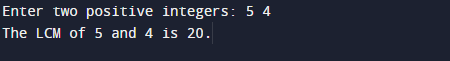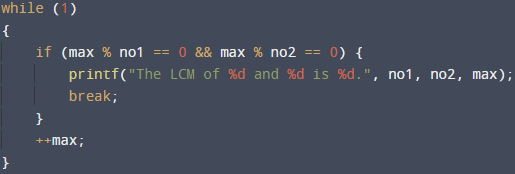C Program to Find LCM of Two Numbers

In this tutorial you will learn about the C Program to Find LCM of Two Numbers and its application with practical example.

In this tutorial, we will learn to create a C program that  will find LCM of two number   using C programming.

Prerequisites

Before starting with this tutorial we assume that you are best aware of the following C programming topics:

• Operators.
• Loop statements
• While loop.
• if else Statement.

What Is LCM?

LCM is a method to finding the smallest possible multiple of two or more numbers. LCM of a numbers is divisible by both the numbers. For example, LCM of 5 and 4 is 20.

C Program to Find LCM of Two Numbers

In this program we will find LCM of two gievn number using while loop. We would first declared and initialized the required variables. Next, we would prompt user to input two numbers.Later we will find the LCM of given number.

OutputIn the above program, we have first declared and initialized a set variables required in the program.

• no1 =It will hold first number given by user.
• no2 =It will hold second number given by user.
• max=This will find biggest among the number.

Let see the step by step method of finding LCM.

LCM of two number, is as follows:
1: user  will be prompted to enter two the positive integer values no1 and no2.
2: Storing the biggest value of no1 & no2 into the max variable.
3: On checking condition whether the max is divisible by both variables no1 and no2 loop  will iterate until it will find a value which divisible by both number.
4: If variable max is divisible,Then display max as the LCM of these two numbers.
5: Else, the value of max is increased, and go to step 3 again and again.
6: Stop the program.LCM stands for Least Common Multiple (LCM). It’s the smallest possible number that is completely divisible by both integer’s no1 and no2, without leaving the remainder. It is also known as the Lowest Common Multiple. and represented by LCM (a, b)/ lcm(a,b).

In this tutorial we have learn about the C Program to Find LCM of Two Numbers and its application with practical example. I hope you will like this tutorial.